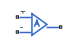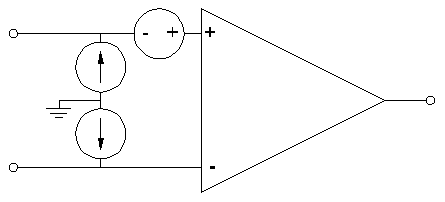# Finite-Gain Op-Amp

Gain-limited operational amplifier model with optional noise

• Library:
• Simscape / Electrical / Integrated Circuits

•## Description

The Finite-Gain Op-Amp block models a gain-limited operational amplifier. If the voltages at the positive and negative ports are Vp and Vm, respectively, the output voltage is:

where:

• A is the gain.

• Rout is the output resistance.

• Iout is the output current.

The input current is:

`$\frac{{V}_{p}-{V}_{m}}{{R}_{in}}$`

where Rin is the input resistance.

The output voltage is limited by the minimum and maximum output values you specify in the block dialog box.

### Thermal Noise

The Finite-Gain Op-Amp block can generate thermal noise. If you set the Noise mode parameter to `Enabled`, then the equivalent circuit for the block includes a noise current source attached to each of the inputs, and a noise voltage source attached to the noninverting input. These three noise sources are independent and uncorrelated.The block generates noise voltage and current according to:

`${v}_{noise}=\frac{{v}_{density}}{\sqrt{2h}}N\left(0,1\right)$`
`${i}_{noise}=\frac{{i}_{density}}{\sqrt{2h}}N\left(0,1\right)$`

where:

• vnoise is the noise voltage.

• vdensity is the single-sided, spectral amplitude density of the voltage noise.

• inoise is the noise current at an input.

• idensity is the single-sided, spectral amplitude density of the current noise applied to that input.

• h is the sampling time.

• N is a Gaussian random number with zero mean and standard deviation of one.

The block generates Gaussian noise by using the PS Random Number source in the Simscape™ Foundation library. You can control the random number seed by setting the Repeatability parameter:

• `Not repeatable` — Every time you simulate your model, the block resets the random seed using the MATLAB® random number generator:

`seed = randi(2^32-1);`
• `Repeatable` — The block automatically generates a seed value and stores it inside the block, to always start the simulation with the same random number. This auto-generated seed value is set when you add a Finite-Gain Op-Amp block from the block library to the model. When you make a new copy of the Finite-Gain Op-Amp block from an existing one in a model, a new seed value is generated. The block sets the value using the MATLAB random number generator command shown above.

• `Specify seed` — If you select this option, additional parameters let you directly specify the random number seed values for input voltage, noninverting input current, and inverting input current.

## Ports

### Conserving

expand all

Electrical conserving port associated with the op-amp noninverting input.

Electrical conserving port associated with the op-amp inverting input.

Electrical conserving port associated with the op-amp output. The port name is hidden on the block icon, but you can see it in simulation data logs.

## Parameters

expand all

### Main

The open-loop gain of the operational amplifier.

The resistance at the input of the operational amplifier that the block uses to calculate the input current.

The resistance at the output of the operational amplifier that the block uses to calculate the drop in output voltage due to output current.

The lower limit on the operational amplifier output voltage.

The upper limit on the operational amplifier output voltage.

### Noise

Select whether to model thermal noise effects:

• `Disabled` — Op-amp does not generate thermal noise.

• `Enabled` — Op-amp generates thermal noise voltage and current, and the associated parameters become visible in the Noise section.

Single-sided, spectral amplitude density of the voltage noise applied to the noninverting input.

#### Dependencies

Enabled when the Noise mode parameter is set to `Enabled`.

Select whether current density values applied to block inputs are different or the same.

#### Dependencies

Enabled when the Noise mode parameter is set to `Enabled`.

Single-sided, spectral amplitude density of the current noise applied to both inputs. Note that even though the density function is the same for both inputs, the actual noise current also depends on the random number seed. If the seeds used for the random number generation are different, then the actual noise currents at the inputs are also different.

#### Dependencies

Enabled when the Noise current parameterization parameter is set to ```Apply same density function to both inputs```.

Single-sided, spectral amplitude density of the current noise applied to noninverting input.

#### Dependencies

Enabled when the Noise current parameterization parameter is set to ```Apply different density function to each input```.

Single-sided, spectral amplitude density of the current noise applied to inverting input.

#### Dependencies

Enabled when the Noise current parameterization parameter is set to ```Apply different density function to each input```.

Defines the rate at which the noise source is sampled. Choose it to reflect the frequencies of interest in your model. Making the sample time too small will unnecessarily slow down your simulation.

#### Dependencies

Enabled when the Noise mode parameter is set to `Enabled`.

Select the noise control option:

• `Not repeatable` — The random sequence used for noise generation is not repeatable.

• `Repeatable` — The random sequence used for noise generation is repeatable, with a system-generated seed.

• `Specify seed` — The random sequence used for noise generation is repeatable, and you control the seed by using the seed parameters. You specify seed values separately for input noise voltage, noninverting input noise current, and inverting input noise current.

#### Dependencies

Enabled when the Noise mode parameter is set to `Enabled`.

Random number seed stored inside the block to make the random sequence repeatable. The parameter value is automatically generated using the MATLAB random number generator command. You can modify this parameter value, but it gets overwritten by a new random value if you copy the block to another block in the model. Therefore, if you want to control the seed of the random sequence, use the ```Specify seed``` option for the Repeatability parameter and specify the desired seed value using the Input noise voltage seed parameter.

#### Dependencies

Enabled when the Repeatability parameter is set to `Repeatable`.

Random number seed stored inside the block to make the random sequence repeatable. The parameter value is automatically generated using the MATLAB random number generator command. You can modify this parameter value, but it gets overwritten by a new random value if you copy the block to another block in the model. Therefore, if you want to control the seed of the random sequence, use the ```Specify seed``` option for the Repeatability parameter and specify the desired seed value using the Noninverting input noise current seed parameter.

#### Dependencies

Enabled when the Repeatability parameter is set to `Repeatable`.

Random number seed stored inside the block to make the random sequence repeatable. The parameter value is automatically generated using the MATLAB random number generator command. You can modify this parameter value, but it gets overwritten by a new random value if you copy the block to another block in the model. Therefore, if you want to control the seed of the random sequence, use the ```Specify seed``` option for the Repeatability parameter and specify the desired seed value using the Inverting input noise current seed parameter.

#### Dependencies

Enabled when the Repeatability parameter is set to `Repeatable`.

Seed used by the noise random number generator.

#### Dependencies

Enabled when the Repeatability parameter is set to `Specify seed`.

Seed used by the noise random number generator.

#### Dependencies

Enabled when the Repeatability parameter is set to `Specify seed`.

Seed used by the noise random number generator.

#### Dependencies

Enabled when the Repeatability parameter is set to `Specify seed`.

## Version History

Introduced in R2008b